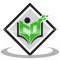## Solve the following equations and check your results.$5 t-3=3 t-5$.Updated on 10-Oct-2022 13:47:38
Given:$5 t-3=3 t-5$.To do: We have to solve the given equation and check the result.Solution:$5t-3=3t-5$$5t-3t=-5+3$$2t=-2$$t=\frac{-2}{2}$$t=-1$Substituting the value of $t$ in LHS, we get,$5t-3=5(-1)-3$$=-5-3$$=-8$Substituting the value of $t$ in RHS, we get,$3t-5=3(-1)-5$$=-3-5$$=-8$LHS $=$ RHSThe value of $t$ is $-1$.

## Solve the following equations and check your results.$3 x=2 x+18$.Updated on 10-Oct-2022 13:47:38

## Solve the following equations.$14 y-8=13$.Updated on 10-Oct-2022 13:47:38

## Solve the following equations.$\frac{2 x}{3}=18$.Updated on 10-Oct-2022 13:47:38

## Solve the following equations.$6 x=12$.Updated on 10-Oct-2022 13:47:38
Given:$6 x=12$.To do: We have to solve the given equation.Solution:$6 x=12$We get,$x=\frac{12}{6}$$x=2$.The value of $x$ is $2$.